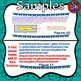# Digital Escape Room-Word Problems 3.0: Real-World, Multi-Step, & Multi-OperationSubject
Resource Type
File Type

PDF

(2 MB)
Product Rating
Standards
Also included in:
1. Use this CCSS aligned, paperless, no-prep, interactive Digital Escape Room-Word Problems BUNDLE:  Addition, Subtraction, Multiplication, and Division on Google Forms as highly engaging assessments, exit slips, test prep, year-end reviews, or interactive additional practice. What a great way to check
\$15.00
\$8.00
Save \$7.00
2. There is no better way to prepare for upcoming standardized testing like I-Learn than implementing the products in this bundle. Included in this Math Test Prep BUNDLE: Word Problems are (5) Digital Escape Rooms on Google Forms, (3) sets of Interactive Digital Task Cards on Google Slides, and (6) se
\$32.50
\$23.40
Save \$9.10
• Product Description
• StandardsNEW

Use this CCSS aligned, paperless, no-prep, interactive Digital Escape Room-Word Problems 3.0:  Addition, Subtraction, Multiplication, and Division on Google Forms as a highly engaging assessment, exit slip, test prep, year-end review, or interactive additional practice. What a great way to check for understanding while students have a blast!!! New features have been added to this escape room to make it even better for students--i.e. funny memes and funny animated G.I.F.s to boost engagement and a new section on the Google Form for each of the six rooms. Students will absolutely love this new format to my digital escape room.  This Escape Room on Google Forms will be the easiest you have ever implemented as it can be completed by students using Chromebooks, computers, laptops,I-Pads, etc. via Google Forms.

There are six rooms to escape in which students must perform tasks and decode a secret message prior to moving on to the next room. Within the rooms there are 42 life-like, real-world, math word problems, a vast majority of which are multi-step, where students have to add, subtract, multiply, and divide.  Prior to moving to the next room they decode a secret word using a code to escape to the next one.

The rooms include the following:

Room 1--Students will add and subtract fractions, convert improper fractions into mixed numbers, convert units of measurement, and calculate volume in multi-step, real-world, word problems.

Room 2--Students will multiply decimals and whole numbers, convert units of measurement, and convert improper fractions into mixed numbers, in multi-step, real-world, word problems.

Room 3--Students will multiply and divide decimals and determine the volume of a figure in multi-step, real-world, word problems.

Room 4--Students will multiply, divide, and subtract decimals and determine the volume of a figure in multi-step, real-world, word problems.

Room 5--Students will add and subtract fractions, convert improper fractions into mixed numbers, convert units of measurement, multiply and add decimals, and divide whole numbers in multi-step, real-world, word problems.

Room 6--Students will divide whole numbers and decimals, subtract decimals, determine the volume of a figure, and determine area of figures in multi-step, real-world, word problems.

After typing in the secret code to this room, students have to type in all the words to determine the secret math message to finally ESCAPE!

Specific directions with screen shots on how to save the Google Form to your drive, how to assign the form to students, and how to see student responses are included. Also included are specific directions which the students will use to complete the Digital Escape Room-Word Problems 3.0:  Addition, Subtraction, Multiplication, and Division on Google Forms.  Lastly, a printable answer key is also included.

Not only is this the easiest Escape Room you will ever use, but also it is a fun way for students to show their math skills.

Find the area of a rectangle with fractional side lengths by tiling it with unit squares of the appropriate unit fraction side lengths, and show that the area is the same as would be found by multiplying the side lengths. Multiply fractional side lengths to find areas of rectangles, and represent fraction products as rectangular areas.
Apply and extend previous understandings of multiplication to multiply a fraction or whole number by a fraction.
Interpret a fraction as division of the numerator by the denominator (𝘢/𝘣 = 𝘢 ÷ 𝘣). Solve word problems involving division of whole numbers leading to answers in the form of fractions or mixed numbers, e.g., by using visual fraction models or equations to represent the problem. For example, interpret 3/4 as the result of dividing 3 by 4, noting that 3/4 multiplied by 4 equals 3, and that when 3 wholes are shared equally among 4 people each person has a share of size 3/4. If 9 people want to share a 50-pound sack of rice equally by weight, how many pounds of rice should each person get? Between what two whole numbers does your answer lie?
Solve word problems involving addition and subtraction of fractions referring to the same whole, including cases of unlike denominators, e.g., by using visual fraction models or equations to represent the problem. Use benchmark fractions and number sense of fractions to estimate mentally and assess the reasonableness of answers. For example, recognize an incorrect result 2/5 + 1/2 = 3/7, by observing that 3/7 < 1/2.
Add and subtract fractions with unlike denominators (including mixed numbers) by replacing given fractions with equivalent fractions in such a way as to produce an equivalent sum or difference of fractions with like denominators. For example, 2/3 + 5/4 = 8/12 + 15/12 = 23/12. (In general, 𝘢/𝘣 + 𝘤/𝘥 = (𝘢𝘥 + 𝘣𝘤)/𝘣𝘥.)
Total Pages
N/A
Included
Teaching Duration
N/A
Report this Resource to TpT
Reported resources will be reviewed by our team. Report this resource to let us know if this resource violates TpT’s content guidelines.\$2.40
List Price:
\$3.00
You Save:
\$0.60